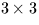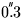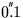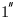Next: SLA_PRENUT - Precession/Nutation Matrix
Up: SUBPROGRAM SPECIFICATIONS
Previous: SLA_PRECES - Precession

## SLA_PRECL - Precession Matrix (latest)

ACTION:
Form the matrix of precession between two epochs, using the model of Simon et al. (1994), which is suitable for long periods of time.

CALL:
CALL sla_PRECL (EP0, EP1, RMATP)

GIVEN:

 D EP0 beginning epoch EP1 D ending epoch

RETURNED:

 D(3,3) RMATP precession matrix

NOTES:
1.
The epochs are TDB Julian epochs.
2.
The matrix is in the sense:
v1 = Mv0
where v1 is the star vector relative to the mean equator and equinox of epoch EP1, M is thematrix RMATP and v0 is the star vector relative to the mean equator and equinox of epoch EP0.
3.
The absolute accuracy of the model is limited by the uncertainty in the general precession, aboutper 1000 years. The remainder of the formulation provides a precision of 1 milliarcsecond over the interval from 1000AD to 3000AD,from 1000BC to 5000AD andfrom 4000BC to 8000AD.

REFERENCE:
Simon, J.L. et al., 1994. Astr.Astrophys. 282, 663.Next: SLA_PRENUT - Precession/Nutation Matrix
Up: SUBPROGRAM SPECIFICATIONS
Previous: SLA_PRECES - Precession

SLALIB --- Positional Astronomy Library